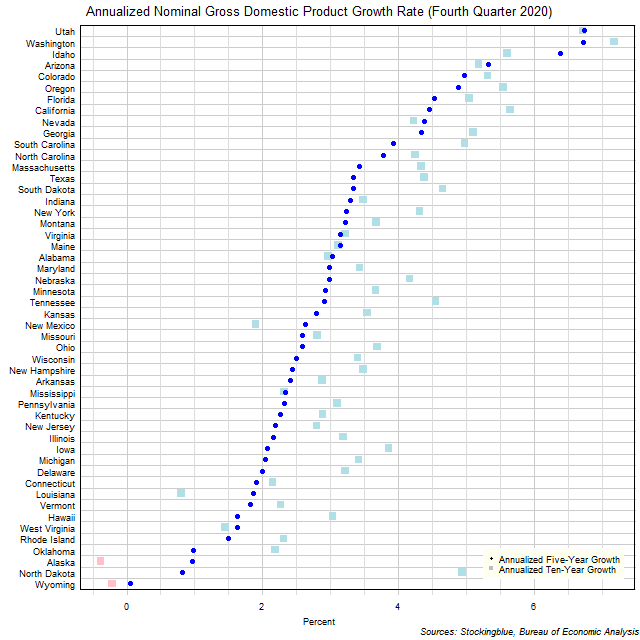# Long-Term US GDP Growth Rate by State, Fourth Quarter 2020

Jun 2, 2021The chart above shows the annualized nominal gross domestic product (GDP) growth rate in each US state over the past five years as of the fourth quarter of 2020 and the growth rate over the past ten years.  Two states experienced negative growth either in the past ten years.

## Findings

• The difference between the state with the largest annualized five-year growth rate, Utah, and the state with the smallest, Wyoming, is 6.68 percentage points.
• The difference between the state with the largest annualized ten-year growth rate, Washington, and the state with the smallest, Alaska, is 7.55 percentage points.
• The median annualized five-year growth rate in the 50 US states is 2.85% and the mean 2.99%.
• The median annualized ten-year growth rate in the 50 US states is 3.48% and the mean 3.59%.
• Of the 50 states, 12 grew faster over the past five years than they did over the past ten years, 0 had the same annualized growth rate over both periods, and 38 grew slower over the past five years than they did over the past ten years.

## Caveats

• Data is from the fourth quarters of 2010, 2015, and 2020.
• The data is seasonally adjusted in current dollars.
• Growth rates may differ from those provided by the Bureau of Economic Analysis as the BEA's growth rates are based on chained dollars in conjunction with the chain index or the quality index for real GDP.
• All figures are rounded to the nearest hundredth.

## Details

Wyoming had the worst performance over the past five years with an annualized growth rate of 0.05%.  Utah had the best performance with an annualized gain of 6.73%.

Over the past ten years, Alaska had the worst performance with an annualized growth rate of -0.38% while Washington had the best performance with an annualized growth rate of 7.17%.

North Dakota had the worst change in its growth rate between its annualized ten-year growth and its annualized five-year growth slowing its rate by 4.12 percentage points.  Alaska had the best change in its growth rate between its annualized ten-year growth and its annualized five-year growth raising its rate by 1.34 percentage points.

New Mexico went from having the 46th largest annualized growth rate over the past ten years to the 27th largest annualized growth rate over the past five years.  Conversely, North Dakota went from having the 11th highest annualized growth rate over the past ten years to the 49th highest annualized growth rate over the past five years.  Three states had annualized growth rates in the top five both over the past ten years and over the past five years: Utah (2nd highest annualized growth over ten years, highest annualized growth over five years), Washington (highest annualized growth over ten years, 2nd highest annualized growth over five years), and Idaho (4th highest annualized growth over ten years, 3rd highest annualized growth over five years).  Two states have ranked in the bottom five over both periods: Alaska (50th ten-year, 48th five-year) and Wyoming (49th ten-year, 50th five-year).

## Sources

US Bureau of Economic Analysis.  2021.  "GDP by State."  Accessed May 7, 2021.  https://www.bea.gov/data/gdp/gdp-state.

Filed under: Charts and Graphs